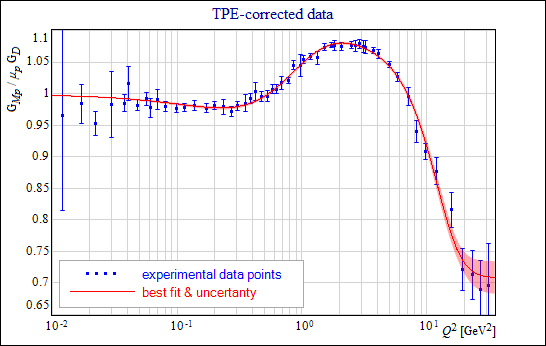### Manual

Krzysztof M. Graczyk, Piotr Plonski, Robert Sulej

### Neural Network Parameterizations of Electromagnetic Nucleon Form Factors

#### Parameterizations of Electromagnetic Nucleon Form Factors - numerical results

Bayesian Framework - go back to the method description

GraNet - neural network library in C++ by K. M. Graczyk

Best fit, in terms of the maximal evidence, is shown for each form factor. The fit uncertainties shown here has been computed using the Hessian approximation formula. The exact and finite differences formulas can be applied to calculations of fit uncertainties with the corresponding project files. Results for each form factor are available in a separate zip archive. Included files are following:

• gXX_data.dat - ascii file, experimental data with uncertainties (constraint point is included at the file end), each row consists of: Q2, G(Q2), σG(Q2);
• gXX_fit.dat - fit numerical results, file format is like for gXX_data.dat;
• gXX.NetAscii - neural network description in NetMaker format (XML file with the network weights, the optimal regularization factor value α used for training, the log evidence, and the network error on training data χ2/N);
• gXX.NetPrj - NetMaker project set up for calculating the fit values from the included network file or recreating the network from scratch.
• where XX is:
• mn for GMn/μnGD
• mp for GMp/μpGD
• ep for GEp/GD
• en for GEn
• the constraint points are following:
• GMn/μnGD (0) = 1 ± 0.001
• GMp/μpGD (0) = 1 ± 0.001
• GEp/GD (0) = 1 ± 0.001
• GEn (0) = 0 ± 0.001
• Files with "_new" sufix correspond to TPE-corrected data (two photon exchange effect). Data in files with "_old" suffix is not TPE-corrected.

#### GMn/μnGD, neutron, magnetic form factor:#### GMp/μpGD, proton, magnetic form factor:#### GEp/GD, proton, electric form factor: